a shot of dev knowledge

RELATED TAGS

# What is the AES algorithm?

## Background

In the 1990s, DES was rendered insecure due to its relatively small 56-bit key size. So, in 1997, the Advanced Encryption Standard (AES) was proposed in response to a public call for proposals by the National Institute of Standards and Technology (NIST). The table below shows how AES compares to its predecessor.

DES AES
Developed 1977 2000
Cipher Type Symmetric block cipher Symmetric block cipher
Block size 64 bits 128 bits
Key length 56 bits 128/192/256 bits
Security Rendered insecure Considered secure

## What is the AES algorithm?

The AES algorithm (also known as the Rijndael algorithm) is a symmetrical block cipher algorithm that takes plain text in blocks of 128 bits and converts them to ciphertext using keys of 128, 192, and 256 bits. Since the AES algorithm is considered secure, it is in the worldwide standard.## How does AES work?

The AES algorithm uses a substitution-permutation, or SP network, with multiple rounds to produce ciphertext. The number of rounds depends on the key size being used. A 128-bit key size dictates ten rounds, a 192-bit key size dictates 12 rounds, and a 258-bit key size has 14 rounds. Each of these rounds requires a round key, but since only one key is inputted into the algorithm, this key needs to be expanded to get keys for each round, including round 0.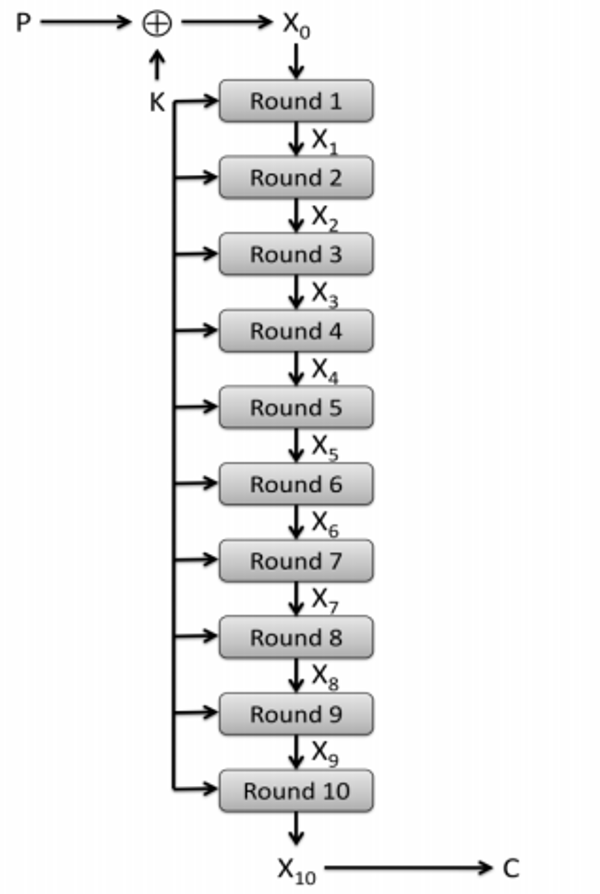Figure 2

## Steps in each round

Each round in the algorithm consists of four steps.

1. Substitution of the bytes
In the first step, the bytes of the block text are substituted based on rules dictated by predefined S-boxes (short for substitution boxes).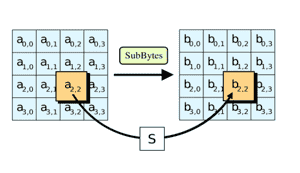2. Shifting the rows
Next comes the permutation step. In this step, all rows except the first are shifted by one, as shown below.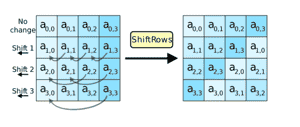3. Mixing the columns
In the third step, the Hill cipher is used to jumble up the message more by mixing the block’s columns.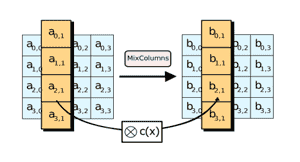In the final step, the message is XORed with the respective round key.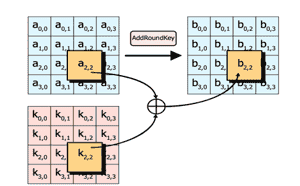When done repeatedly, these steps ensure that the final ciphertext is secure.

## AES modes

The AES algorithm has five modes of operation, namely:

RELATED TAGS

RELATED COURSES

View all Courses

Keep Exploring

Learn in-demand tech skills in half the time